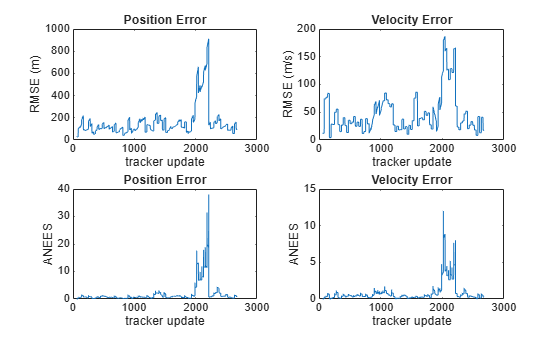# cumulativeTrackMetrics

Cumulative metrics for recent tracks

## Syntax

``metricsTable = cumulativeTrackMetrics(errorMetrics)``

## Description

example

````metricsTable = cumulativeTrackMetrics(errorMetrics)` returns a table of cumulative metrics, `metricsTable`, for every track identifier provided in the most recent update.```

## Examples

collapse all

Examine the assignments and errors for a system tracking two targets.

First, load the stored track data.

`load trackmetricex tracklog truthlog`

Create objects to analyze assignment and error metrics.

```tam = trackAssignmentMetrics; tem = trackErrorMetrics;```

Create the output variables.

```posRMSE = zeros(numel(tracklog),1); velRMSE = zeros(numel(tracklog),1); posANEES = zeros(numel(tracklog),1); velANEES = zeros(numel(tracklog),1);```

Loop over all tracks to:

• Extract the tracks and ground truth at the i th tracker update.

• Analyze and retrieve the current track-to-truth assignment.

• Analyze instantaneous error metrics over all tracks and truths.

```for i=1:numel(tracklog) tracks = tracklog{i}; truths = truthlog{i}; [trackAM,truthAM] = tam(tracks, truths); [trackIDs,truthIDs] = currentAssignment(tam); [posRMSE(i),velRMSE(i),posANEES(i),velANEES(i)] = ... tem(tracks,trackIDs,truths,truthIDs); end```

Show the track metrics table.

`trackMetricsTable(tam)`
```ans=4×15 table TrackID AssignedTruthID Surviving TotalLength DeletionStatus DeletionLength DivergenceStatus DivergenceCount DivergenceLength RedundancyStatus RedundancyCount RedundancyLength FalseTrackStatus FalseTrackLength SwapCount _______ _______________ _________ ___________ ______________ ______________ ________________ _______________ ________________ ________________ _______________ ________________ ________________ ________________ _________ 1 NaN false 1120 false 0 false 3 3 false 0 0 false 0 0 2 NaN false 1736 false 0 false 8 88 false 0 0 false 28 3 6 3 true 1138 false 0 false 4 314 false 1 28 false 0 2 8 2 true 662 false 0 false 2 29 false 1 169 false 28 0 ```

Show the truth metrics table.

`truthMetricsTable(tam)`
```ans=2×10 table TruthID AssociatedTrackID DeletionStatus TotalLength BreakStatus BreakCount BreakLength InCoverageArea EstablishmentStatus EstablishmentLength _______ _________________ ______________ ___________ ___________ __________ ___________ ______________ ___________________ ___________________ 2 8 false 2678 false 4 168 true true 56 3 6 false 2678 false 3 645 true true 84 ```

Plot the RMSE and ANEES error metrics.

```subplot(2,2,1) plot(posRMSE) title('Position Error') xlabel('tracker update') ylabel('RMSE (m)') subplot(2,2,2) plot(velRMSE) title('Velocity Error') xlabel('tracker update') ylabel('RMSE (m/s)') subplot(2,2,3) plot(posANEES) title('Position Error') xlabel('tracker update') ylabel('ANEES') subplot(2,2,4) plot(velANEES) title('Velocity Error') xlabel('tracker update') ylabel('ANEES')```Show the current error metrics for each individual recorded track.

`currentTrackMetrics(tem)`
```ans=2×5 table TrackID posRMS velRMS posANEES velANEES _______ ______ ______ ________ ________ 6 44.712 20.988 0.05974 0.31325 8 129.26 12.739 1.6745 0.2453 ```

Show the current error metrics for each individual recorded truth object.

`currentTruthMetrics(tem)`
```ans=2×5 table TruthID posRMS velRMS posANEES velANEES _______ ______ ______ ________ ________ 2 129.26 12.739 1.6745 0.2453 3 44.712 20.988 0.05974 0.31325 ```

Show the cumulative error metrics for each individual recorded track.

`cumulativeTrackMetrics(tem)`
```ans=4×5 table TrackID posRMS velRMS posANEES velANEES _______ ______ ______ ________ ________ 1 117.69 43.951 0.58338 0.44127 2 129.7 42.8 0.81094 0.42509 6 371.35 87.083 4.5208 1.6952 8 130.45 53.914 1.0448 0.44813 ```

Show the cumulative error metrics for each individual recorded truth object.

`cumulativeTruthMetrics(tem)`
```ans=2×5 table TruthID posRMS velRMS posANEES velANEES _______ ______ ______ ________ ________ 2 258.21 65.078 2.2514 0.93359 3 134.41 48.253 0.96314 0.49183 ```

## Input Arguments

collapse all

Error metrics object, specified as a `trackErrorMetrics` System object™.

## Output Arguments

collapse all

Track error metrics, returned as a table.

• When you set the `ErrorFunctionFormat` property of the input error metrics object to `'built-in'`, the table columns depend on the setting of the `MotionModel` property.

 Motion Model Table Columns `'constvel'` `posRMSE`, `velRMSE`, `posANEES`, `velANEES` `'constacc'` `posRMSE`, `velRMSE`, `accRMSE`, `posANEES`, `velANEES`, `accANEES` `'constturn'` `posRMSE`, `velRMSE`, `yawRateRMSE`, `posANEES`, `velANEES`, `yawRateANEES`

RMSE and ANEES denote root mean squared error and average normalized estimation error squared of a track for the entire tracking scenario time history. For example, the cumulative position RMSE value for a track is defined as:

`$\text{posRMSE}=\sqrt{\frac{1}{N}\sum _{t=1}^{N}‖\Delta {p}_{t}{‖}^{2}}$`

where N is the total number of time steps that the track has an associated truth.

`$\Delta {p}_{t}={p}_{track,t}-{p}_{truth,t}$`

is the difference between the position of the track at time step t, ptrack,t, and the position of the associated truth at time step t, ptruth,t. The cumulative RMSE values for other states (`vel`, `pos`, `acc`, and `yawRate`) are defined similarly. The position ANEES value, `posANEES` is defined as:

`$posANEES=\frac{1}{N}\sum _{t=1}^{N}\Delta {p}_{t}{}^{T}{C}_{p,t}^{-1}\Delta {p}_{t}$`

where Cp,t is the covariance corresponding to the position of the track at time step t. The ANEES values for other states (`vel`, `pos`, `acc`, and `yawRate`) are defined similarly.

• When you set the `ErrorFunctionFormat` property to `'custom'`, the table contains the arithmetically averaged values of the custom metrics output from the error function.

Introduced in R2018b